# 基于深度学习识别模型的缺陷检测

## 二、缺陷数据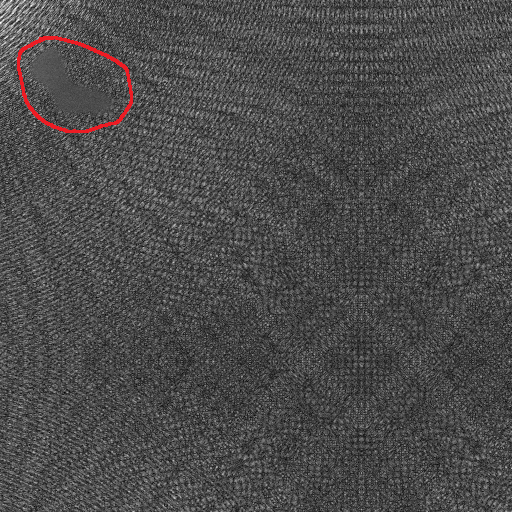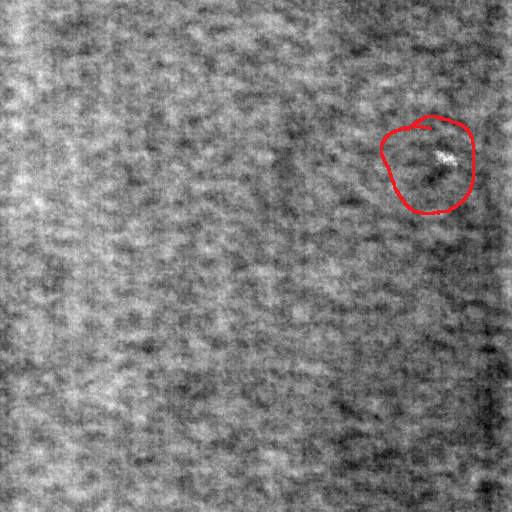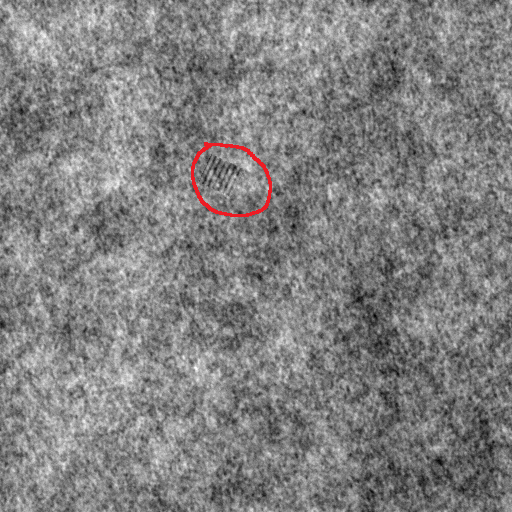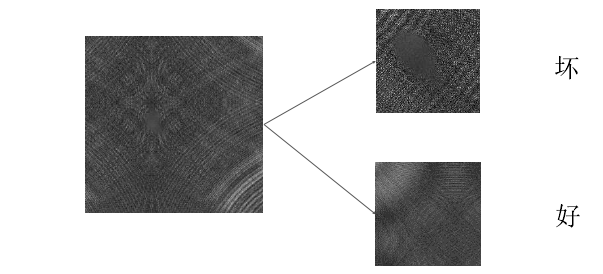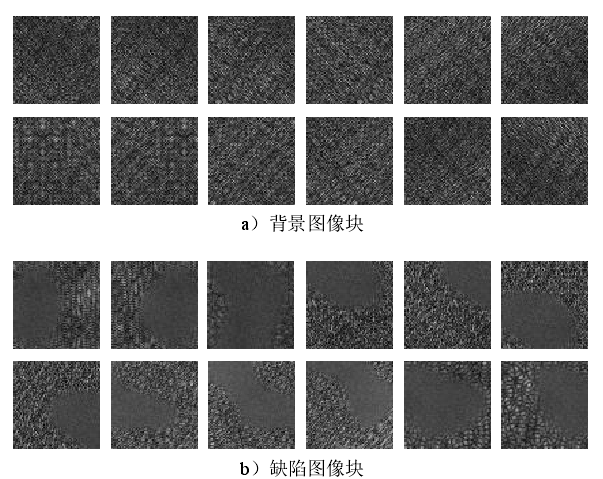## 三、网络结构

Conv1：64x3x3
Conv2：128x3x3
ResNetBlock和DenseNetBlock各两个，具体细节请参考残差网络和DenseNet。
Conv3：128x3x3
Maxpool：stride=2，size=2x2
FC1：4096
Dropout1：0.5
FC2：1024
Dropout1：0.5
Softmax：对应的就是要分的类别，在这里我是二分类。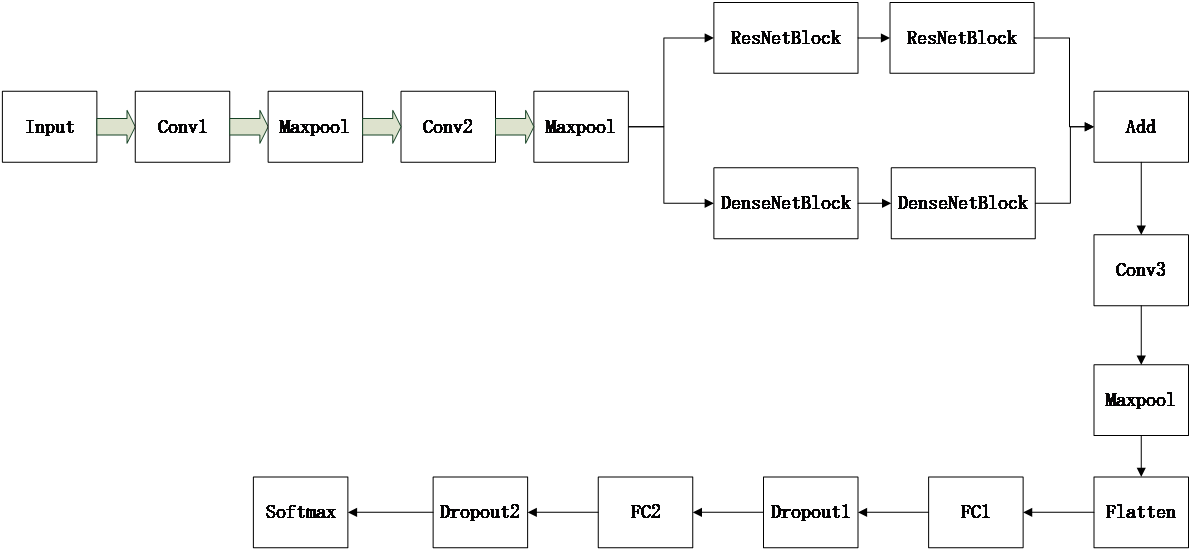def focal_loss(y_true, y_pred):
pt_1 = tf.where(tf.equal(y_true, 1), y_pred, tf.ones_like(y_pred))
return -K.sum(K.pow(1. - pt_1, 2) * K.log(pt_1))


## 四、整幅场景图像的缺陷检测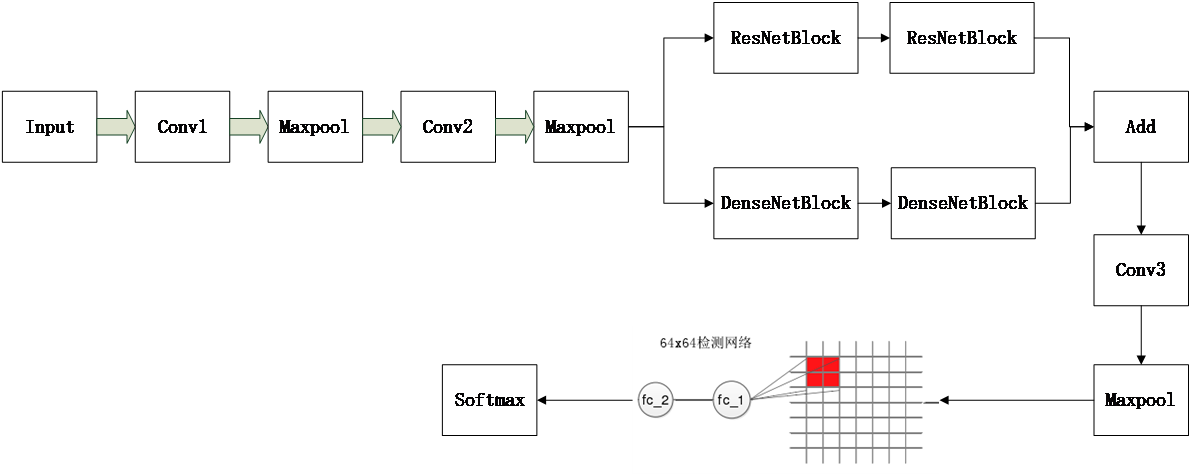#提取特征的大模型
# 第一个卷积和最大池化层
X = Conv2D(16, (3, 3), name="conv2d_1")(inputs)
X = BatchNormalization(name="batch_normalization_1")(X)
X = Activation('relu', name="activation_1")(X)
X = MaxPooling2D(pool_size=(2, 2), strides=(2, 2), name="max_pooling2d_1")(X)
conv_1 = Conv2D(32, (1, 1), padding='same', name='conv2d_2')(X)
conv_1 = BatchNormalization(name='batch_normalization_2')(conv_1)
conv_1 = Activation('relu', name='activation_2')(conv_1)
conv_2 = Conv2D(32, (3, 3), padding='same', name='conv2d_3')(X)
conv_2 = BatchNormalization(name='batch_normalization_3')(conv_2)
conv_2 = Activation('relu', name='activation_3')(conv_2)
conv_3 = Conv2D(32, (5, 5), padding='same', name='conv2d_4')(X)
conv_3 = BatchNormalization(name='batch_normalization_4')(conv_3)
conv_3 = Activation('relu', name='activation_4')(conv_3)
pooling_1 = MaxPooling2D(pool_size=(2, 2), strides=(1, 1), padding='same', name='max_pooling2d_2')(X)
X = merge([conv_1, conv_2, conv_3, pooling_1], mode='concat', name='merge_1')
X = MaxPooling2D(pool_size=(2, 2), strides=(2, 2), name='max_pooling2d_3')(X)  # 这里的尺寸变成16x16x112
X = Conv2D(64, (3, 3), kernel_regularizer=regularizers.l2(0.01), padding='same', name='conv2d_5')(X)
X = BatchNormalization(name='batch_normalization_5')(X)
X = Activation('relu', name='activation_5')(X)
X = MaxPooling2D(pool_size=(2, 2), strides=(2, 2), name='max_pooling2d_4')(X)  # 这里尺寸变成8x8x64
X = Conv2D(128, (3, 3), padding='same', name='conv2d_6')(X)
X = BatchNormalization(name='batch_normalization_6')(X)
X = Activation('relu', name='activation_6')(X)
X = MaxPooling2D(pool_size=(2, 2), strides=(2, 2), padding='same', name='max_pooling2d_5')(X)  # 这里尺寸变成4x4x128

return X

X_ = Flatten(name='flatten_1')(inputs_sec)
X_ = Dense(256, activation='relu', name="dense_1")(X_)
X_ = Dropout(0.5, name="dropout_1")(X_)
predictions = Dense(2, activation='softmax', name="dense_2")(X_)
return predictions
#建立的小模型

inputs=Input(shape=(512,512,3))
#建立第一个model
model=Model(inputs=inputs, outputs=X)


## 五、识别定位结果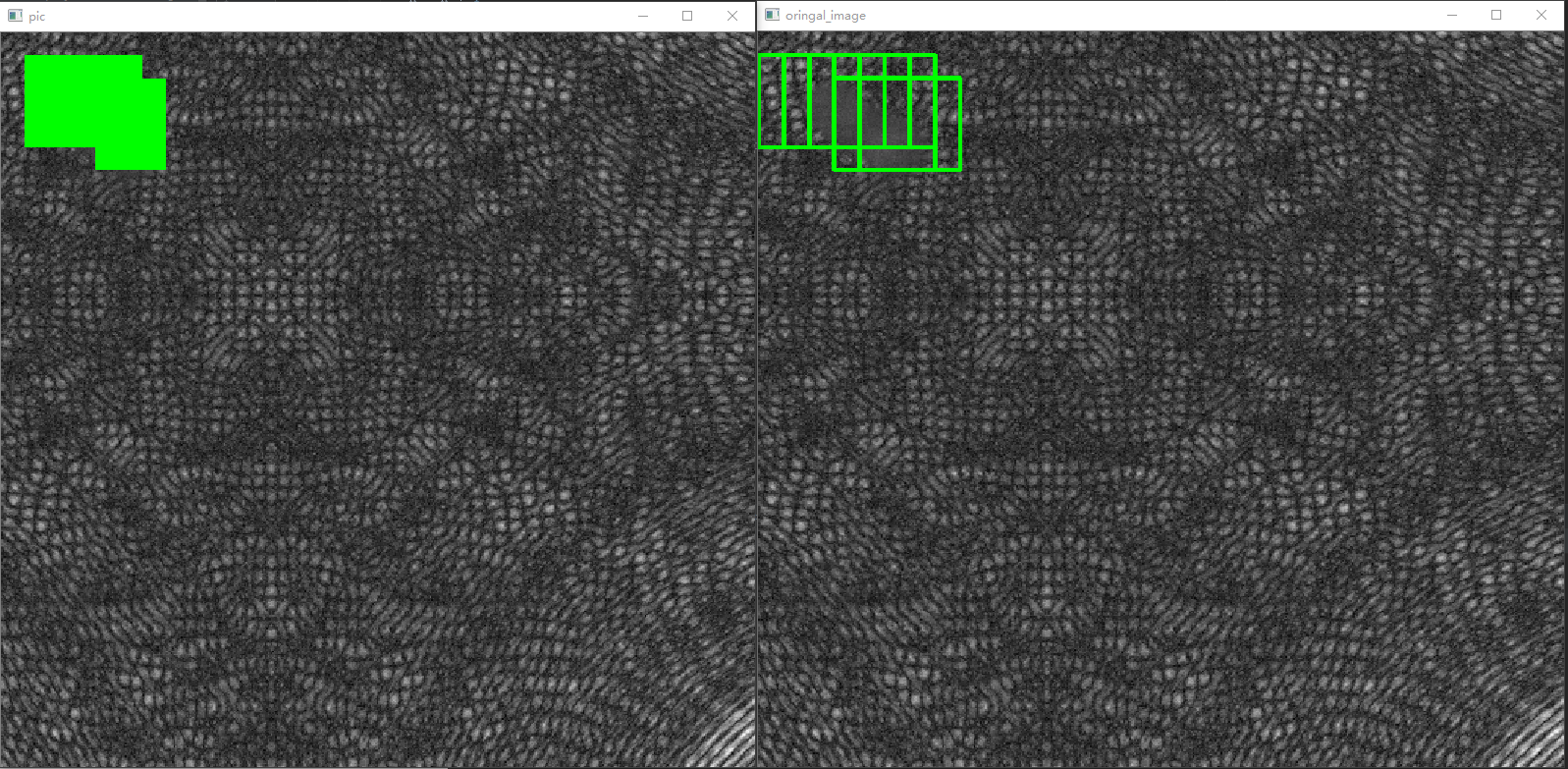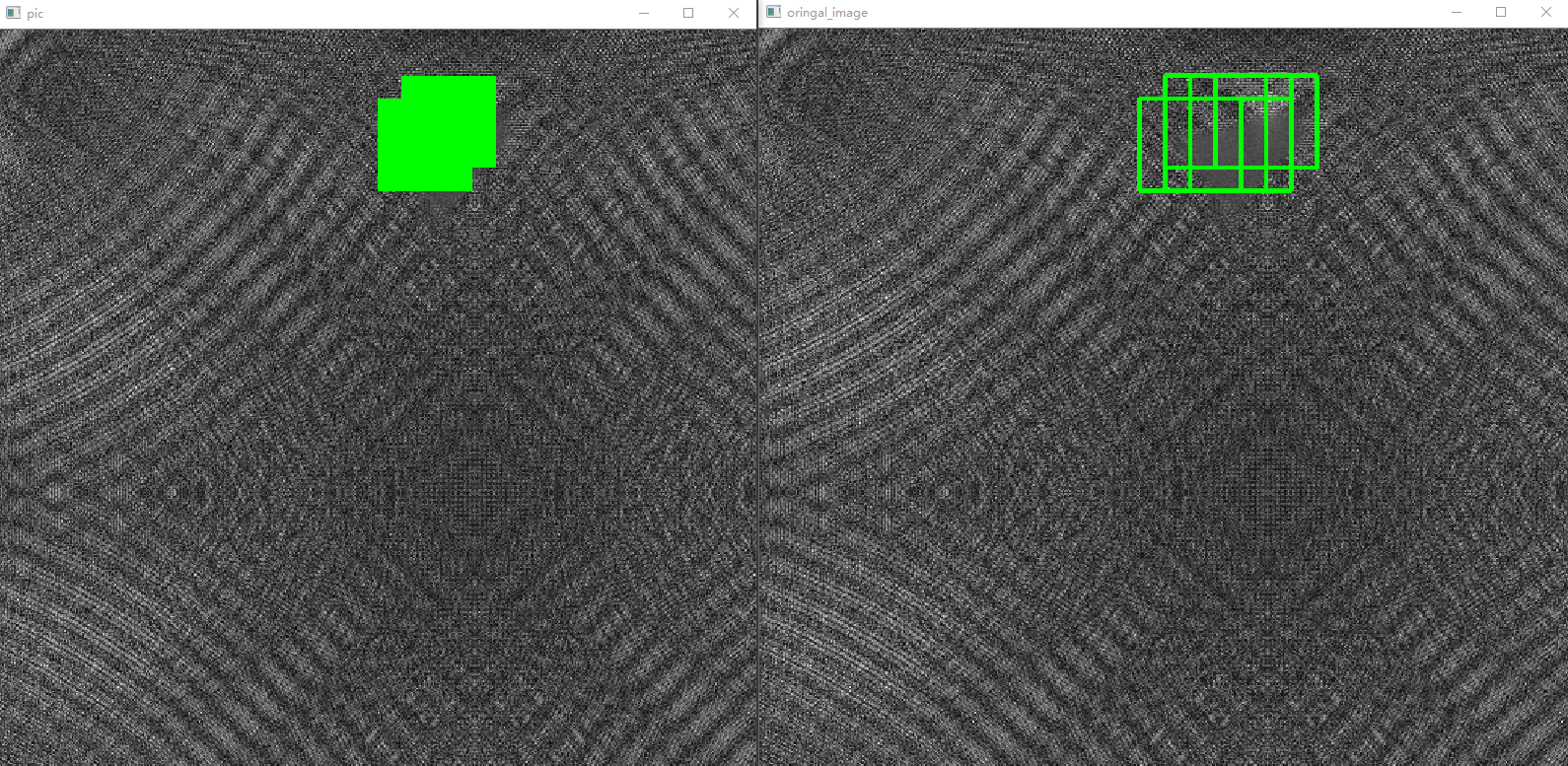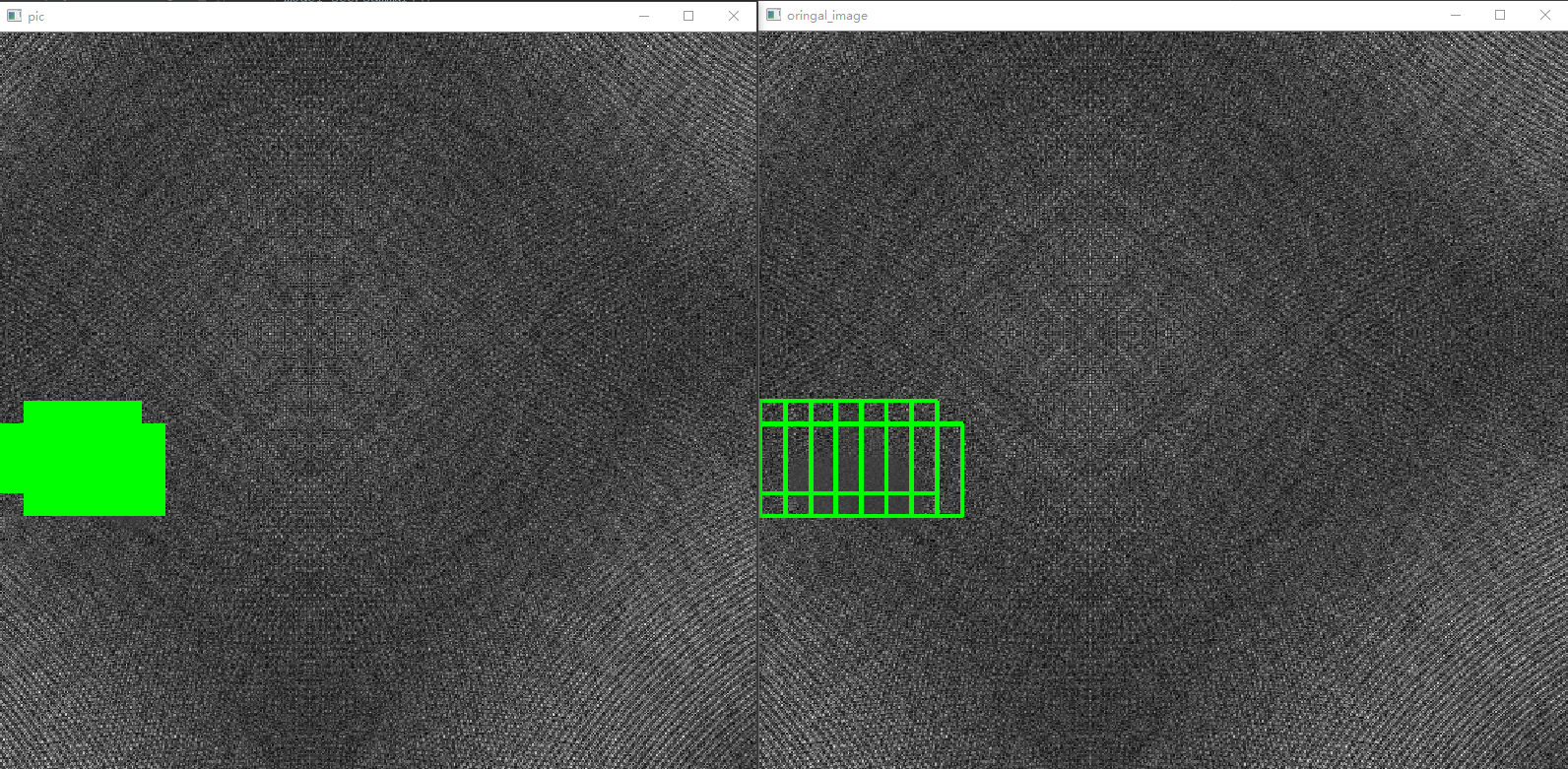## 七、关于源代码©️2019 CSDN 皮肤主题: 编程工作室 设计师: CSDN官方博客